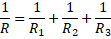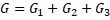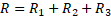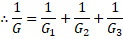# Conductance and Resistivity

Conductance, Temperature coefficient, resistivity and specific resistance are the properties of an electric conductor or any other material. They all can be understood by resistance. Resistance is the amount of obstruction in the flow of current. Means resistance is the obstruction in the flow of electrons. All these properties of the material can be understood by resistance and also all these properties are dependent on the value of resistance of that material.

## Properties of Conductor

#### Conductance

Conductance is an expression of the ease with which electric current flows through a material. Thus, conductance is a measure of how easy it is for electrons to flow through a material. As you all know resistance is the obstruction in the flow of electrons. So mathematically, conductance is the reciprocal of resistance.

Conductance = 1 / Resistance

The greater will be the resistance, the less the conductance and smaller will be the resistance, greater would be the conductance. If the resistance of material A is half than material B. Then, material A has double conductance than material B.

The symbol of conductance is capital “G” and the unit is 1 / ohm or and it is more popularly known as mho which is ohm spelled backward. Some years ago, mho was also replaced by a new unit Siemens.

Now if we want to find the conductance of series and parallel combination of resistors, it’s a quite easy job now.

For the parallel combination of resistors,And you know that G = 1/R. So, the total conductance would beThus, total conductance of resistors in parallel combination can be expressed as the addition of conductance of each individual resistor.

For the series combination of resistors,And you know that G = 1/R and so R = 1/G.Therefore in the series combination, reciprocal of total conductance is the addition of reciprocal of the entire individual conductance.

#### Resistivity

Resistivity is a measure of the resistance of a given size of a specific material to electrical conduction. Resistivity is the measure of how strongly a material opposes the flow of electric current. Resistivity is also reciprocal of conductance. More will be the resistivity, less amount of current will pass through the conductor and thus amount of conductance would be less and less the resistivity, more amount of current will pass through the conductor and conductance would be more.

SI unit of resistivity is ohm meter. It is represented by symbol ρ.

Materials that conduct electrical current easily are called conductors and they have low resistivity. Those that do not conduct electric current are said to be insulators and they have high resistivity.

Formula of resistivity

As you all know from one of the laws of resistance, R = ρ l/a where ρ is the resistivity. So we can derive the formula of resistivity

ρ = R a/l

Thus resistivity is directly proportional to the cross-sectional area of the material and inversely proportional to the length of the material.

ρ α a/l

Thus resistivity is same as resistance but it is the measure of resistance. Also, the formula and dependency of resistivity are different than resistance. Resistance is directly dependent on the length and inversely proportional to the area but resistivity is directly dependent on the cross-sectional area and inversely proportional to the length of the material.

#### Specific Resistance

The resistance of the conductor is directly proportional to the length of the material and inversely proportional to the cross-sectional area of the material.

R α l/a

So if the length of the material increases, the value of resistance also increases and if the area is decreased, the value of resistance increases. Thus, the value of resistance will change if there are changes in length and cross-sectional area of the material. Specific resistance is a constant type of resistance being calculated for material at specific length area. Value of specific resistance of any material never changes.

Symbol of specific resistance is the same as resistivity and that is ρ. And also the SI unit of specific resistance is ohm – meters which is the same as the unit of resistivity. Also, mathematically specific resistance is given by the formula

ρ = R a/l

The specific resistances of some materials are given in the table above. They are all calculated at 20 degree Celsius. And they are represented with a unit of ohms – cmil/ft. However, the SI unit for specific resistance is ohm-meter (Ω-m) with 1.66243 × 10-9 Ω – meters per Ω – cmil/ft. Values of specific resistance of any material are usually scaled at µ Ω – cm.

#### Temperature Coefficient of Resistance

As you know the electric current is nothing but an array of electrons which under electromotive force atoms revolve in a conductor. But, still, there is some influence of temperature on the electrons generation. If a metal like copper is subjected to heat, the electrons are energized and their resistance increases with rising in temperature. This feature is often known as temperature coefficient of resistance.

It is defined as the increase of electrical resistance per degree rise in temperature for every ohm of resistance. The temperature coefficient is positive when the resistance increases (known as the positive temperature coefficient of resistance). Similarly, it is negative when the resistance decreases (also known as the negative temperature coefficient of resistance).

The below table shows the conductivity, specific resistance and temperature coefficient of some metals. From the first column of the table, it can be seen that copper and silver has less resistance, while Manganin and constantan have high resistance. The remaining metals are used for making resistances, whereas copper and silver are used as conductors.

MetalConductivitySpecific ResistanceTemperature Coefficient
Copper58.20.01720.0039
Silver62.50.0160.0038
Aluminium33.30.030.0036
Iron8.330.120.0045
Tin8.330.120.0045
Zinc15.90.0530.0037